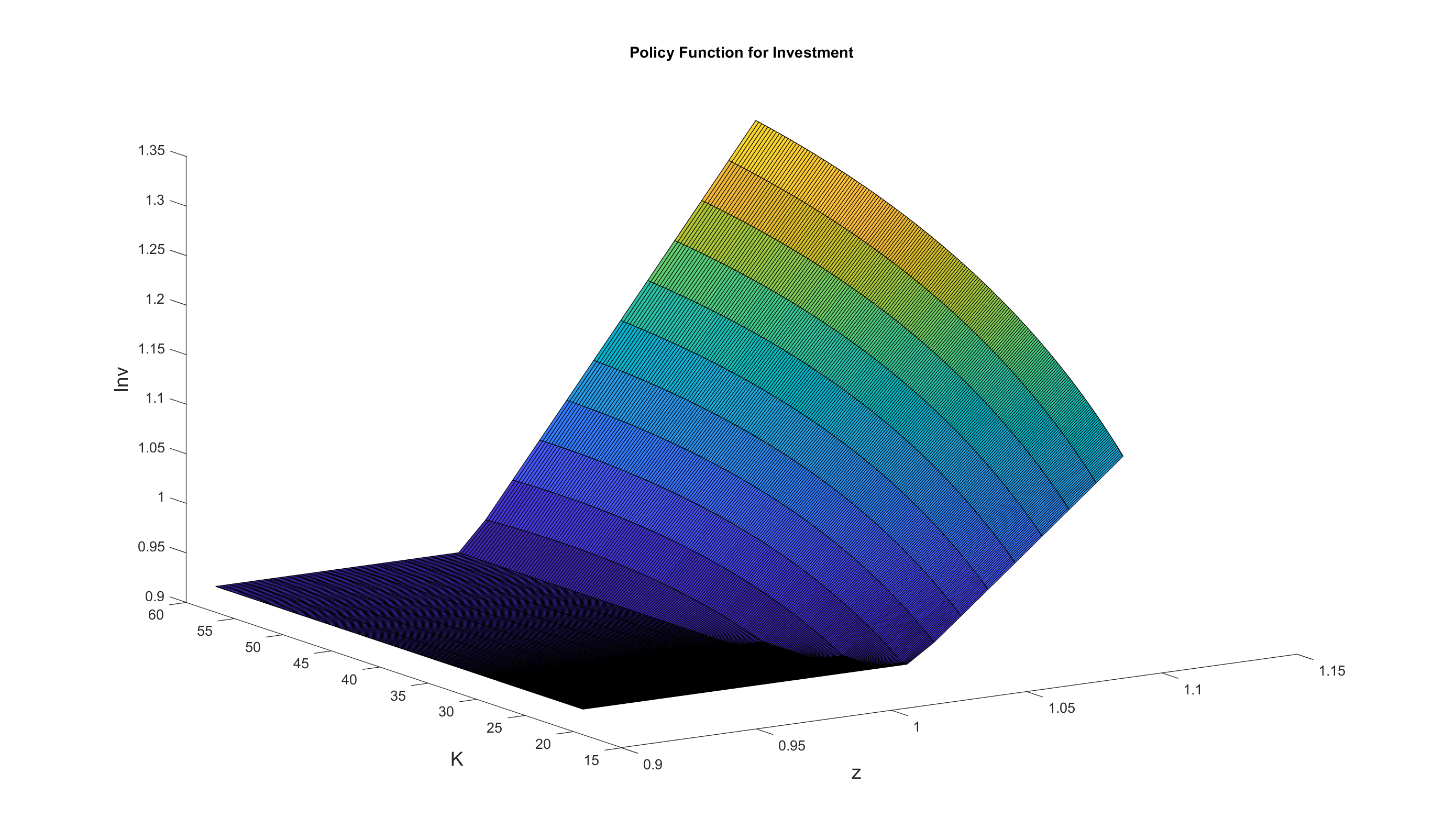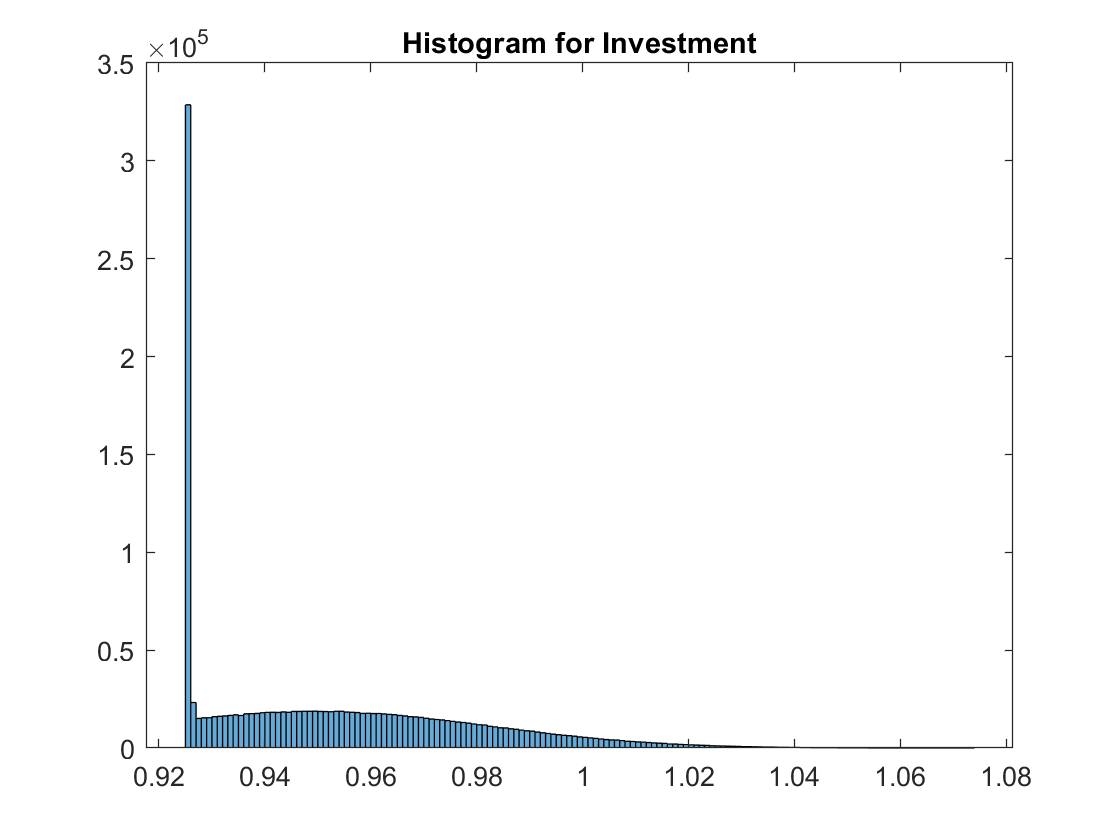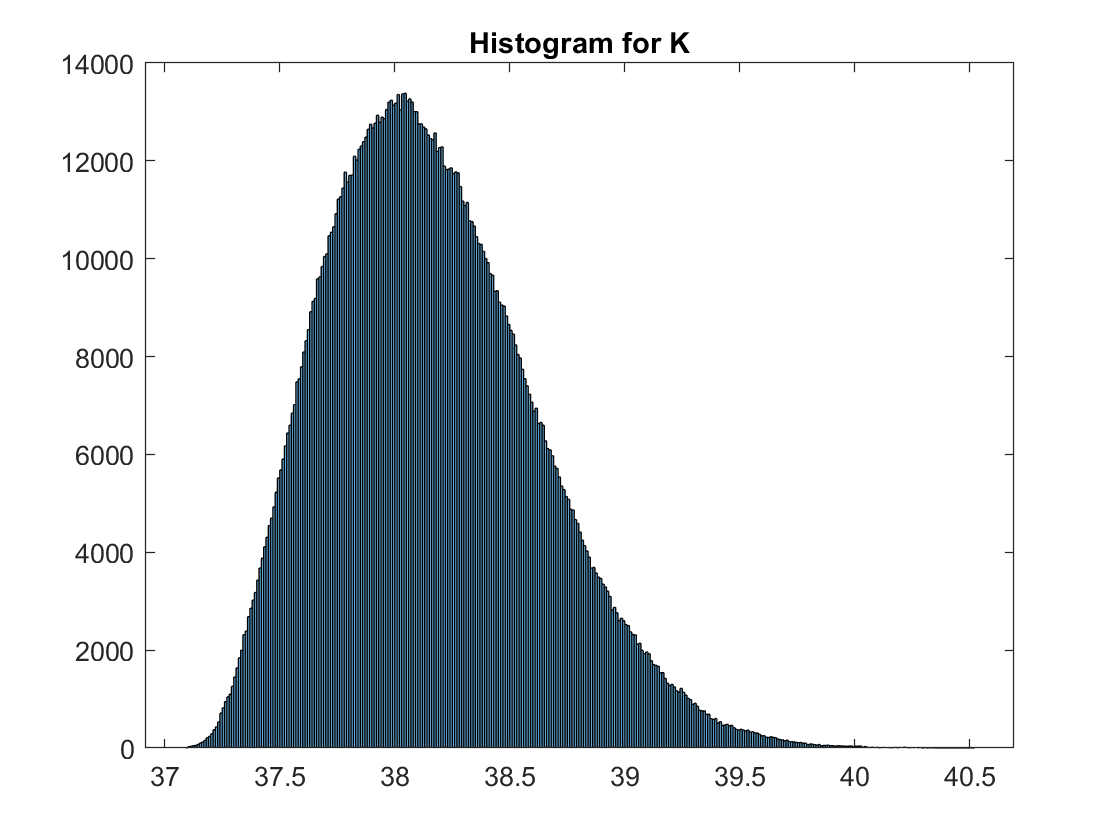# An RBC Model with Irreversible Investment

The standard RBC model can also be solved easily using local methods. Now we consider an extension which can only be solved properly using global methods. The model features an investment irreversibility constraint, which requires investment to be larger than a certain threshold.

## The Model

We augment the simple RBC model with two additional features.

First, productivity follows a continuous AR(1) process

$\log z_t = \rho \log z_{t-1} + \epsilon_t$

where parameter $$\rho \in (0,1)$$ is the persistence, $$\epsilon_t$$ is an I.I.D. shock.

Second, when accumulating capital,

$K_{t+1} = K_t(1-\delta) + I_t,$

the representative firm is subject to an irreversibility constraint

$I_t \geq \phi I_{ss},$

where $$I_{ss}$$ is the steady-state level of investment and parameter $$\phi$$ corresponds to the tightness of the irreversibility constraint.

These features require us to keep track of two continuous state variables: exogenous $$z_t$$ and endogenous $$K_t$$. The toolbox is well designed to handle models with more than one continuous state variables like the current one.

Let $$\mu_t$$ denote the Lagrange multiplier on the irreversibility constraint, the complete-markets equilibrium can be characterized by the Euler equation, the complementary-slackness condition, and the goods market clearing condition:

$\begin{split}&c_t^{-\sigma}-\mu_t\\ &=\beta \mathbb{E}_t \left[\left ( \alpha z_{t+1} K_{t+1}^{\alpha-1}+(1-\delta)\right)c_{t+1}^{-\sigma}-(1-\delta)\mu_{t+1}\right],\\ &\\ &\mu_t (K_{t+1}-(1-\delta)K_t-\phi I_{ss}) = 0,\\ &\\ &c_t+K_{t+1} = z_t K_t^{\alpha}+(1-\delta)K_t.\end{split}$

A recursive competitive equilibrium consists of functions: $$c(z,K), K'(z,K), \mu(z,K)$$ s.t.

$\begin{split}&c(z,K)^{-\sigma}-\mu(z,K)\\ &=\beta \mathbb{E}\left[ \left (\alpha z' [K'(z,K)]^{\alpha-1}+(1-\delta)\right)[c(z',K'(z,K))]^{-\sigma}-(1-\delta)\mu(z',K'(z,K)) \Big| z \right]\\ &\\ &\mu(z,K)(K'(z,K)-(1-\delta)K-\phi I_{ss})=0\\ &\\ &c(z,K)+K'(z,K)=zK^{\alpha} + (1-\delta)K.\end{split}$

This recursive system can be solved using a time iteration procedure similar to the one for the standard RBC model. In the period-$$t$$ time step, we take functions $$c_{t+1}(z,K),\mu_{t+1}(z,K)$$ as known, and solve for $$c_t,\mu_t,K'_t$$ from the system of three equations:

$\begin{split}&c_t(z,K)^{-\sigma}-\mu_t(z,K)\\ &=\beta \mathbb{E}\left[ \left (\alpha z' [K_t'(z,K)]^{\alpha-1}+(1-\delta)\right)[c_{t+1}(z',K_t'(z,K))]^{-\sigma}-(1-\delta)\mu_{t+1}(z',K_t'(z,K))\Big| z \right]\\ &\\ &\mu_t(z,K)(K'_t(z,K)-(1-\delta)K-\phi I_{ss})=0\\ &\\ &c_t(z,K)+K'_t(z,K)=zK^{\alpha} + (1-\delta)K.\end{split}$

We keep iterating until convergence.

## The gmod File

The recursive system can now be input to the GDSGE toolbox via a mod file rbcIrr.gmod below.

  1% Parameters
2parameters beta sigma alpha delta K_min K_max rho Imin;
3
4beta = 0.99; % dicount factor
5sigma = 2; % risk aversion
6alpha = 0.36; % capital share
7delta = 0.025; % depreciation rate
8rho = 0.90; %persistent of productivity shocks
9phi = 0.975; %investment irreversibility constraint
10
11TolEq = 1e-6;
12SaveFreq = 50;
13SimuSaveFreq = 1000;
14SimuPrintFreq = 1000;
16
17% Shocks
18var_shock e;
19shock_num = 2;
20shock_trans = [1/2.0,1/2.0;1/2.0,1/2.0];
21e = [-0.01,0.01]*sqrt(1-rho^2);
22
24Kss = (alpha/(1/beta - 1 + delta))^(1/(1-alpha));
25Iss = delta*Kss;
26Imin = phi*Iss;
27K_min = 0.5*Kss;
28K_max = 1.5*Kss;
29
30% States
31var_state z K;
32z_pts = 21;
33z = linspace(0.9,1.1,z_pts);
34K_pts = 201;
35K = exp(linspace(log(K_min),log(K_max),K_pts));
36
37var_interp Euler_interp muc_interp;
38
39% Last period
40var_policy_init c;
41inbound_init c 1e-6 100;
42
43var_aux_init Euler mu muc;
44
45model_init;
46  Y = z*(K^alpha);
47  resid = 1 - c/(Y-Imin);
48
49  mu = 1;
50  muc = mu*(c^-sigma);
51  Euler = (c^-sigma)*(1-delta+z*alpha*K^(alpha-1));
52
53  equations;
54     resid;
55  end;
56end;
57
58initial Euler_interp Euler;
59Euler_interp = Euler;
60initial muc_interp muc;
61muc_interp = muc;
62
63% Endogenous variables, bounds, and initial values
64var_policy c invst mu;
65
69
70% Other equilbirium variables
71var_aux Y Euler K_next muc z_next Inv;
72
73model;
74  %investment irreversiility constraint
75  Inv = invst + Imin;
76  K_next = Inv + (1-delta)*K;
77  Y = z*(K^alpha);
78  resid  = 1 - (Inv+c)/Y;
79
80  %transition to z_next
81  z_next' = exp(rho*log(z)+e');
82
83  %interpolation of future policies
84  Euler_future' = Euler_interp'(z_next',K_next);
85  EEuler_future = GDSGE_EXPECT{Euler_future'};
86  muc_future' = muc_interp'(z_next',K_next);
87  Emuc_future = GDSGE_EXPECT{muc_future'};
88
89  Euler = (c^-sigma)*(1-delta+z*alpha*K^(alpha-1));
90  muc = mu*(c^-sigma);
91
92  % First order conditions for firms
93  foc = 1-mu - (beta*EEuler_future-beta*(1-delta)*Emuc_future)/(c^-sigma);
94  comp_slack = mu*invst;
95
96  equations;
97      foc;
98      resid;
99      comp_slack;
100  end;
101end;
102
103simulate;
104  num_periods = 15000;
105  num_samples = 100;
106
107  initial K Kss;
108  initial z 1;
109  initial shock 2;
110
111  var_simu z K Y c Inv;
112  K' = K_next;
113  z' = z_next';
114end;


The toolbox solves the model and produces the policy functions. The following figure displays the investment policy function $$I(z,K)$$. The investment irreversibility constraint tends to bind when $$K$$ is low or $$z$$ is low.We then use the policy functions to simulate the model. The following figures show the long run distributions of investment and capital. The investment irreversibility constraint binds around 20% of the times. The distribution of capital is asymmetric and skewed towards lower levels of capital. It is significantly different from the distribution in the RBC model without the irreversibility constraint.